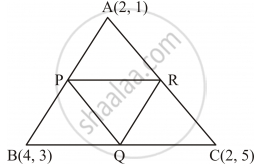Advertisement Remove all ads

# Find the Area of Triangle Formed by Joining the Mid-points of the Sides of the Triangle Whose Vertices Are A(2, 1), B(4, 3) and C(2, 5). - Mathematics

Advertisement Remove all ads
Advertisement Remove all ads
Advertisement Remove all ads

Find the area of triangle formed by joining the mid-points of the sides of the triangle whose vertices are A(2, 1), B(4, 3) and C(2, 5).

Advertisement Remove all ads

#### Solution

Let ABC be the triangle such that A (2, 1), B (4, 3) and C (2, 5) are the vertices of the triangle.Let P, Q and R be the mid-points of sides AB, BC and CA respectively of ΔABC.

\text{Coordination of the mid-point of points}(x_1,y_2) and (x_2,y_2)=((x_1+x_2)/2, (y_1+y_2)/2)

\text{Coordination of P}=((4+2)/2, (3+1)/2)=(3,2)

\text{Coordination of Q}=((4+2)/2, (3+5)/2)=(3,4)

\text{Coordination of Q}=((2+2)/2, (5+1)/2)=(2,3)

The area of the triangle whose vertices are (x1 , y1 ), (x2 , y2 ) and (x3 , y3 ) is given as follows:

1/2[x_1(y_2-y_3)+x_2(y_3-y_1)+x_3(y_1-y_2)]

∴Area of ΔPQR

=1/2[3(4-3)+3(3-2)+2(2-4)]

=1/2[3(1)+3(1)+2(-2)]

=1/2[3+3-4]

=2/2

=1 sq.unit

Thus, the area of the triangle formed by joining the mid-points of the sides of the given triangle

is 1 sq unit.

Concept: Areas of Combinations of Plane Figures
Is there an error in this question or solution?

#### APPEARS IN

Advertisement Remove all ads
Share
Notifications

View all notifications

Forgot password?